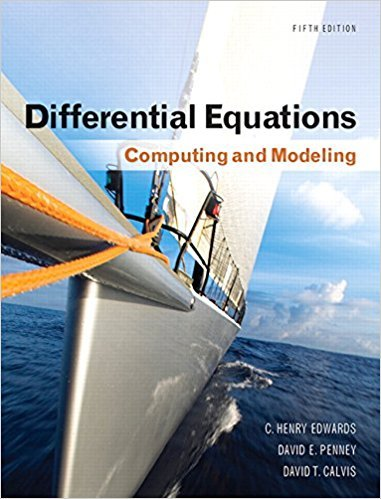×
Log in to StudySoup
Get Full Access to Math - Textbook Survival Guide
Join StudySoup for FREE
Get Full Access to Math - Textbook Survival Guide

Already have an account? Login here
×
Reset your password

Textbooks / Math / Differential Equations: Computing and Modeling 5

# Differential Equations: Computing and Modeling 5th Edition - Solutions by Chapter## Full solutions for Differential Equations: Computing and Modeling | 5th Edition

ISBN: 9780321816252Differential Equations: Computing and Modeling | 5th Edition - Solutions by Chapter

Solutions by Chapter
4 5 0 360 Reviews
##### ISBN: 9780321816252

The full step-by-step solution to problem in Differential Equations: Computing and Modeling were answered by , our top Math solution expert on 01/24/18, 05:45AM. Differential Equations: Computing and Modeling was written by and is associated to the ISBN: 9780321816252. This expansive textbook survival guide covers the following chapters: 6. Since problems from 6 chapters in Differential Equations: Computing and Modeling have been answered, more than 2503 students have viewed full step-by-step answer. This textbook survival guide was created for the textbook: Differential Equations: Computing and Modeling, edition: 5.

Key Math Terms and definitions covered in this textbook
• Exponential eAt = I + At + (At)2 12! + ...

has derivative AeAt; eAt u(O) solves u' = Au.

• Fast Fourier Transform (FFT).

A factorization of the Fourier matrix Fn into e = log2 n matrices Si times a permutation. Each Si needs only nl2 multiplications, so Fnx and Fn-1c can be computed with ne/2 multiplications. Revolutionary.

• Fourier matrix F.

Entries Fjk = e21Cijk/n give orthogonal columns FT F = nI. Then y = Fe is the (inverse) Discrete Fourier Transform Y j = L cke21Cijk/n.

• Kronecker product (tensor product) A ® B.

Blocks aij B, eigenvalues Ap(A)Aq(B).

• Length II x II.

Square root of x T x (Pythagoras in n dimensions).

• Linear combination cv + d w or L C jV j.

Vector addition and scalar multiplication.

• Linearly dependent VI, ... , Vn.

A combination other than all Ci = 0 gives L Ci Vi = O.

• Multiplicities AM and G M.

The algebraic multiplicity A M of A is the number of times A appears as a root of det(A - AI) = O. The geometric multiplicity GM is the number of independent eigenvectors for A (= dimension of the eigenspace).

• Outer product uv T

= column times row = rank one matrix.

• Pascal matrix

Ps = pascal(n) = the symmetric matrix with binomial entries (i1~;2). Ps = PL Pu all contain Pascal's triangle with det = 1 (see Pascal in the index).

• Polar decomposition A = Q H.

Orthogonal Q times positive (semi)definite H.

• Random matrix rand(n) or randn(n).

MATLAB creates a matrix with random entries, uniformly distributed on [0 1] for rand and standard normal distribution for randn.

• Row space C (AT) = all combinations of rows of A.

Column vectors by convention.

• Singular matrix A.

A square matrix that has no inverse: det(A) = o.

• Solvable system Ax = b.

The right side b is in the column space of A.

• Spectral Theorem A = QAQT.

Real symmetric A has real A'S and orthonormal q's.

• Symmetric matrix A.

The transpose is AT = A, and aU = a ji. A-I is also symmetric.

• Unitary matrix UH = U T = U-I.

Orthonormal columns (complex analog of Q).

• Vandermonde matrix V.

V c = b gives coefficients of p(x) = Co + ... + Cn_IXn- 1 with P(Xi) = bi. Vij = (Xi)j-I and det V = product of (Xk - Xi) for k > i.

• Wavelets Wjk(t).

Stretch and shift the time axis to create Wjk(t) = woo(2j t - k).